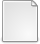# The Combinatorics of Tandem Duplication

Penso-Dolfin, L, Wu, Taoyang and Greenman, C (2015) The Combinatorics of Tandem Duplication. Discrete Applied Mathematics, 194. 1–22. ISSN 0166-218XOther (pdf) - Submitted VersionPreview
PDF (greenman_DAM) - Submitted Version
Tandem duplication is an evolutionary process whereby a segment of DNA is replicated and proximally inserted. The different configurations that can arise from this process give rise to some interesting combinatorial questions. Firstly, we introduce an algebraic formalism to represent this process as a word producing automaton. The number of words arising from n tandem duplications can then be recursively derived. Secondly, each single word accounts for multiple evolutions. With the aid of a bi-coloured 2d- tree, a Hasse diagram corresponding to a partially ordered set is constructed, from which we can count the number of evolutions corresponding to a given word. Thirdly, we implement some subtree prune and graft operations on this structure to show that the total number of possible evolutions arising from n tandem duplications is $\prod_{k=1}^n(4^k - (2k + 1))$. The space of structures arising from tandem duplication thus grows at a super-exponential rate with leading order term $\mathcal{O}(4^{\frac{1}{2}n^2})$.View Item